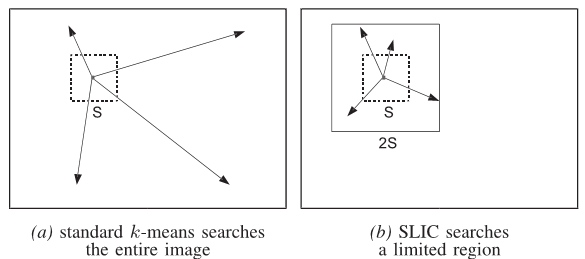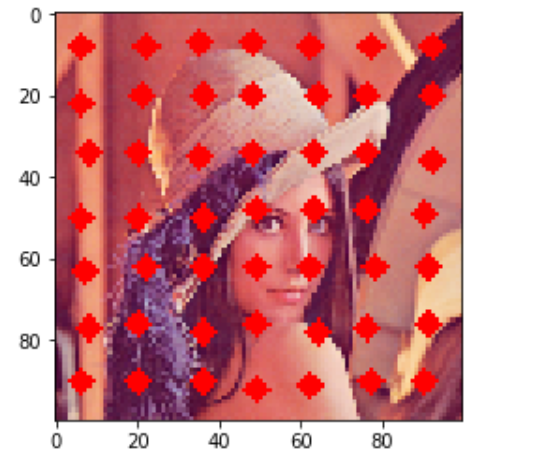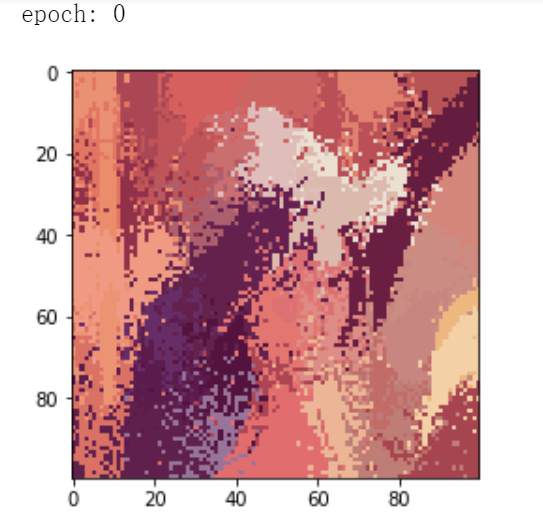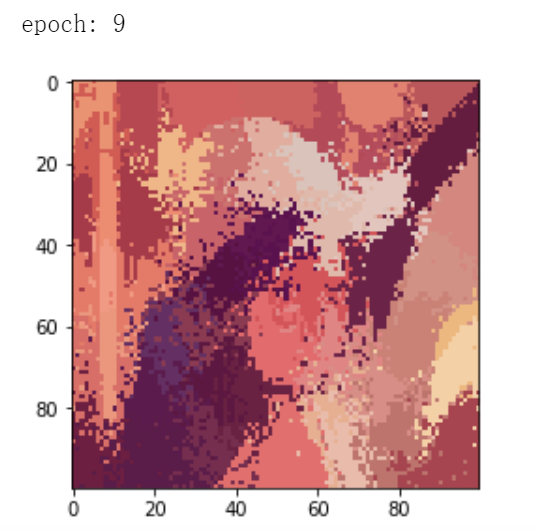•研究论文
• 在Windows系统下，通过设置生成像素数目和参数M，可以对输入影像进行超像素分割，生成超像素分割的jpg影像
• 该程序代码是完整的Matlab代码，实现了超像素分割算法功能。超像素分割能将很小的区域目标准确分割，是现在研究的热点分割算法！这个程序供广大研究者参考！
• 超像素已成为许多计算机视觉系统中必不可少的处理单元，图像的超像素分割是最重要的步骤之一。 本文提出了一种有效的超像素分割算法。 我们为超像素分割（MBS）引入了一种新的紧凑型感知最小障碍距离，并为层次结构...研究论文
• 像素分割为图像分割，图像处理的基础，文件为MATLAB和C混编的代码，demo为主程序，运行demo主程序进行超像素分割
• 像素分割代码 超像素概念是2003年Xiaofeng Ren提出和发展起来的图像分割技术，是指具有相似纹理、颜色、亮度等特征的相邻像素构成的有一定视觉意义的不规则像素块。它利用像素之间特征的相似性将像素分组,用少量的...
• 像素分割软件，让你快速将超像素方法应用于你的图像文件，并输出超像素分割效果，为你文章讲解和计划实验做准备。
• 基于分水岭的超像素分割方法，效率给常高，分割效果也非常不错，适合超像素分割算法的朋友们学习。
• 掌握超像素分割的原理、超像素分割方法的推导过程以及实现方法 1.1 超像素 超像素是指将具有相似纹理、颜色、亮度等特征的相邻像素聚合成某一个像素块。结合超像素的思想，使得少量的像素块可以用来代替原本大量的...
学习目标
掌握超像素分割的原理、超像素分割方法的推导过程以及实现方法
1.1 超像素
超像素是指将具有相似纹理、颜色、亮度等特征的相邻像素聚合成某一个像素块，结合超像素的思想，这样可以使少量的像素块代替原本大量的像素。
目前超像素广泛应用于图像分割、目标识别等领域。
1.2  SLIC
SLIC（Simple Linear IterativeClustering，简单线性迭代聚类)是超像素分割中使用比较多的方法，主要特点（优点）如下：
1.基于LAB颜色空间
2.运行速度快，生成的超像素紧凑
3.思想比较简单
1.3 SLIC实现的具体步骤
1.初始化种子点
在图像内均匀分布种子点（与Kmeans不同）
若图像像素为N*N，预想分为K个像素块，则：

每个像素块的初始大小为$N*N/K$

相邻种子点间的步长S为 $\sqrt {N*N/K}$（假设初始每个像素块的大小是均匀的）

2.重新选择种子点
结合图像中的梯度信息，在n*n邻域内重新选择种子点（一般n取3)。重新选择种子点是为了防止均匀初始化后的种子点处于边缘或是噪声，将种子点移动到梯度比较小的位置。
3.以各像素块种子点为中心更新标签
以超像素的种子点为中心，在其上下左右2S的范围内搜索（和Kmeans 不同，这里的搜索不是全局范围的）4.计算距离
计算各像素点与种子点的距离，距离包括颜色距离与空间位置的距离。
颜色距离$d_c$的计算方法如下：
$\sqrt {l_j-l_i)^2+(a_i-a_j)^2+(b_i-b_j)^2}$
空间距离$d_s$的计算方法如下：
$\sqrt {(x_j-x_i)^2+(y_i-y_j)^2}$
总距离$D$的计算方法如下：
$\sqrt {(\frac{d_c}{m})^2+(\frac{d_s}{S})^2}$
其中$m$为常数，取值范围在[1,40]，常以10代替
因为搜索范围在种子点的[-2S,2S]内，有些像素点会被重复搜索到，所以应该同时记录距离，最终取最小值对应的种子点作为其聚类中心
将上述3-4过程不断迭代，一直到满足最大迭代次数或者中心种子点不再发生变化为止。
5.增强连通性
经过迭代之后有可能会出现过分割、多连通、单个超像素被分割成多个不连续的超像素等，需要增强连通性

主要思路是：新建一张标记表，表内元素均为-1，按照“Z”型走向（从左到右，从上到下顺序）将不连续的超像素、尺寸过小超像素重新分配给邻近的超像素，遍历过的像素点分配给相应的标签，直到所有点遍历完毕为止

2 实战
import cv2
import numpy as np
from skimage import img_as_float
import matplotlib.pyplot as plt

1.初始化种子点
def init_cluster(pic,n_segments):
cluster_w,cluster_h=int(cluster_S/2),int(cluster_S/2)  #计算出每个的长和宽
center={}
i=0
while cluster_h<pic.shape:  #shape是高度
while cluster_w<pic.shape:
center[i]=[cluster_w,cluster_h,pic[cluster_w,cluster_h,0],pic[cluster_w,cluster_h,1],pic[cluster_w,cluster_h,2]]
cluster_w=cluster_w+cluster_S
i=i+1
cluster_w=int(cluster_S/2)
cluster_h=cluster_h+cluster_S
return center

2.计算梯度
def caculate_grad(w,h):
if h+1>=pic.shape or w+1>=pic.shape:
w=w-2
h=h-2

3.在3*3邻域内根据计算得到的梯度，更新种子点
def update_center(center):#更新中心点
for i in range(0,len(center)):
w,h=center[i],center[i]
for dw in range(-1,2): #在3*3邻域内
for dh in range(-1,2):
center[i]=[w+dw,h+dh,pic[w+dw,h+dh,0],pic[w+dw,h+dh,1],pic[w+dw,h+dh,2]]
return center

4.可视化种子点
def draw_center(center)
for i in range(0,len(center)):
cv2.circle(ori_pic,(center[i],center[i]),1, (255, 0, 0),4) #将初始化中心标出来
fig=plt.figure()
ax.imshow(ori_pic)
plt.show()

n_segments=50
ori_pic=cv2.cvtColor(ori_pic,cv2.COLOR_BGR2RGB)
pic=cv2.cvtColor(ori_pic,cv2.COLOR_BGR2LAB)
cluster_shape=pic.shape*pic.shape/n_segments    #每个超像素块中包含的像素数
cluster_S=int(np.sqrt(cluster_shape))  #超像素块的长/宽/初始种子点之间的距离（假设形状规则）
center=init_cluster(pic,n_segments) #初始化中心
center=update_center(center)  #更新中心，避免中心在梯度高（噪声点等）
draw_center(center) #可视化初始中心点

得到初始的种子点（已经进行了梯度更新，可以看出中心点分布不是绝对均匀）5.初始化距离矩阵（用来存储每个像素点与其中心点间的距离）
def init_distance():
distance=[]
for i in range(pic.shape):
distance_item=[np.inf for j in range(pic.shape)]
distance.append(distance_item)
return distance

7.初始化像素矩阵（用来记录每个像素块中包含的具体像素位置）
def init_pixel():
pixel={}
for i in range(0,len(center)):
pixel[i]=[]
return pixel

8.计算某像素与其种子点之间的距离（这里M取10）
def caculate_distance(w_,h_,center_):#根据颜色空间和像素位置进行更新
color_dic=np.sqrt(np.sum(np.square(pic[w_,h_,:]-np.array(center_[2:]))))
geo_dic=np.sqrt(np.sum(np.square(np.array([w_,h_])-np.array(center_[:2]))))
dis=np.sqrt(np.square(color_dic/10)+np.square(geo_dic/cluster_S))
return dis

9.计算各个像素点所属的标签（即所属种子点）
def get_cluster(center,distance,label,pixel):
for i in range(0,len(center)):
for dw in range(center[i]-2*cluster_S,center[i]+2*cluster_S):  #在2S范围内
if dw<0 or dw>=pic.shape:  continue
for dh in range(center[i]-2*cluster_S,center[i]+2*cluster_S):
if dh<0 or dh>=pic.shape:  continue
dis=caculate_distance(dw,dh,center[i])#计算距离
if dis<distance[dw][dh]:
distance[dw][dh]=dis
label[(dw,dh)]=center[i]  #记录当前的中心点
for j in list(pixel.values()):
if(dw,dh) in j:#若该像素点之前已经隶属于某个中心，需要先将其去掉，再添加至新的中心
j.remove((dw,dh))
pixel[i].append((dw,dh))
return label,distance,pixel

10.更新各超像素的中心（所属种子点）
def update_cluster(center,pixel):#更新中心
for i,item in enumerate(pixel.values()): #{1:[(),()]
w,h=0,0
for j in item:
w+=j
h+=j
center_w=int(w/len(item))
center_h=int(h/len(item))
center[i]=[center_w,center_h,pic[center_w,center_h,0],pic[center_w,center_h,1],pic[center_w,center_h,2]]
return center


11.可视化超像素分割结果
def save_cluster(center,pixel):
image_arr = np.copy(ori_pic)
for i,item in enumerate(pixel.values()): #{1:[(),()]
for j in item:
image_arr[j,j,0]=image_arr[center[i],center[i],0]
image_arr[j,j,1]=image_arr[center[i],center[i],1]
image_arr[j,j,2]=image_arr[center[i],center[i],2]
fig=plt.figure()
ax.imshow(image_arr)
plt.show()

label={}
distance=init_distance()
pixel=init_pixel()#初始化簇内的像素点 形如：{0: [], 1: [], 2: [], 3: [], 4: [], 5: []}

for epoch in range(10):#循环迭代十次
old_label=label
print('epoch:',epoch)
label,distance,pixel=get_cluster(center,distance,old_label,pixel)
center=update_cluster(center,pixel)
save_cluster(center,pixel)

最终可以得到超像素分割的结果为：调包:
from skimage.segmentation import slic,mark_boundaries
from skimage import img_as_float
segments = slic(img_as_float(pic), n_segments=50,sigma=2)
marked_img=mark_boundaries(img_as_float(cv2.cvtColor(pic, cv2.COLOR_BGR2RGB)), segments)
fig=plt.figure()
# fig.show(marked_img)
ax.imshow(marked_img)
plt.axis('off')
plt.show()参考文献
 Achanta,Radhakrishna, et al. “SLIC superpixels compared to state-of-the-artsuperpixel methods.” Pattern Analysis and Machine Intelligence, IEEETransactions on 34.11 (2012): 2274-2282.


展开全文• 基于贝叶斯算法的图像像素分割，并可视化分割结果。matlab程序
• 生成的超像素如同细胞一般紧凑整齐，邻域特征比较容易表达。这样基于像素的方法可以比较容易的改造为基于超像素的方法。...相比其他的超像素分割方法，SLIC在运行速度、生成超像素的紧凑度、轮廓保持方面都比较理想。
• 像素分割C代码，修改后不会出现warning C4244: “=”: 从“double”转换到“int”，可能丢失数据 error C2275: “mwSize”: 将此类型用作表达式非法 error C2065: “numdims”: 未声明的标识符 error C2146: 语法...
• 像素分割算法，亲测可用，内含数据图片，不用mex，直接matlab运行
• 分析了超像素分割领域的发展现状，以基于图论的方法和基于梯度下降的方法为视角，对现有超像素分割方法 进行归纳和论述。在此基础上，就目前常用的超像素分割算法进行了实验对比，分析各自的优势和不足。最后， 对超...
• SLIC： simple linear ... 这是一个基于聚类算法的超像素分割，由LAB空间以及x、y像素坐标共5维空间来计算。不仅可以分割彩色图，也可以兼容分割灰度图，它还有一个优点就是可以人为的设置需要分割的超像素的数量。
•研究论文
•研究论文
• 基于SLIC方法的超像素分割算法代码，纯MATLAB编写。
• 线性光谱聚类（LSC）的超像素分割算法，该算法可以生成具有低计算成本的紧凑且均匀的超像素。基本上，基于测量图像像素之间的颜色相似性和空间接近度的相似性度量，采用超像素分割的归一化切割公式。然而，代替使用...matlab
• #%% SEED超像素分割 import cv2 import numpy as np import imageio # print(dir(cv2.ximgproc)) img = imageio.imread(r'E:\Vaihingen\data\orginalimages\top_mosaic_09cm_area31.tif')[:,:,::-1] converted_img ...
#%% SEED超像素分割
import cv2
import numpy as np
import imageio
# print(dir(cv2.ximgproc))

converted_img = cv2.cvtColor(img, cv2.COLOR_BGR2HSV)

# print(type(img_feature))

#seed参数
height,width,channels = converted_img.shape

num_iterations = 15
prior = 3
double_step = True
num_superpixels = 30000
num_levels = 6
num_histogram_bins = 10

# seed
seeds = cv2.ximgproc.createSuperpixelSEEDS(width, height, channels, num_superpixels, num_levels, prior, num_histogram_bins)
seeds.iterate(converted_img,num_iterations)  #输入图像大小必须与初始化形状相同，迭代次数为10
label_seeds = seeds.getLabels()
number_seeds = seeds.getNumberOfSuperpixels()
imageio.imsave('img_seeds.png',img_seeds[:,::,::-1])

结果：展开全文• 首先分析了超像素分割领域的发展现状，以基于图论的方法和基于梯度下降的方法为视角，对现有超像素分割方法进行归纳和论述。在此基础上，就目前常用的超像素分割算法进行了实验对比，分析各自的优势和不足。最后，对...梯度下降...